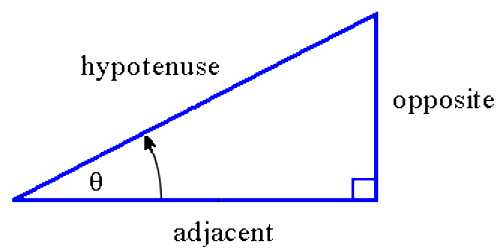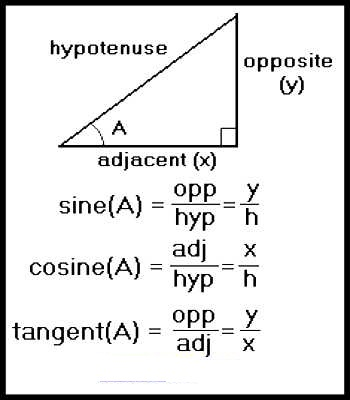Mathematic

# Trigonometry TermsTrigonometry Terms

Trigonometry is a branch of mathematics that studies relationships involving lengths and angles of triangles. The study of angles and of the angular relationships of planar and three-dimensional figures is known as trigonometry. A right triangle consists of one angle of 900 and two acute angles. Each acute angle of a right triangle has the properties of sine, cosine, and tangent. The sine, cosine, and tangent of an acute angle of a right triangle are ratios of two of the three sides of the right triangle.The sine of the angle is the ratio of the length of the side opposite the angle divided by the length of the hypotenuse. It is the trigonometric function that is equal to the ratio of the side opposite a given angle (in a right-angled triangle) to the hypotenuse. In a trigonometric function sin θ that for all real numbers θ is exactly equal to the sine of an angle of measure θ in radians and that is given by the sum of the alternating series

The cosine of the angle is the ratio of the length of the side adjacent to the angle divided by the length of the hypotenuse. It is the trigonometric function that is equal to the ratio of the side adjacent to an acute angle (in a right-angled triangle) to the hypotenuse. In a trigonometric function that for an acute angle is the ratio between the leg adjacent to the angle when it is considered part of a right triangle and the hypotenuse

The tangent of the angle is the ratio of the length of the side opposite the angle divided by the length of side adjacent to the angle. It is a straight line or plane that touches a curve or curved surface at a point, but if extended does not cross it at that point. It is the trigonometric function that for an acute angle is the ratio between the leg opposite to the angle when it is considered part of a right triangle and the leg adjacent.

Information Source;